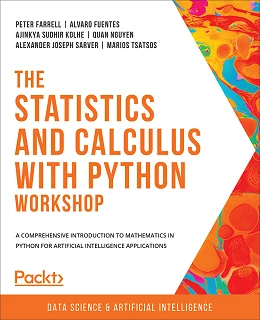# The Statistics and Calculus with Python Workshop### eBook Details:

• Paperback: 740 pages
• Publisher: WOW! eBook (August 18, 2020)
• Language: English
• ISBN-10: 1800209762
• ISBN-13: 978-1800209763

### eBook Description:

The Statistics and Calculus with Python Workshop: With examples and activities that help you achieve real results, applying calculus and statistical methods relevant to advanced data science has never been so easy

Are you looking to start developing artificial intelligence applications? Do you need a refresher on key mathematical concepts? Full of engaging practical exercises, The Statistics and Calculus with Python Workshop will show you how to apply your understanding of advanced mathematics in the context of Python.

The book begins by giving you a high-level overview of the libraries you’ll use while performing statistics with Python. As you progress, you’ll perform various mathematical tasks using the Python programming language, such as solving algebraic functions with Python starting with basic functions, and then working through transformations and solving equations. Later chapters in the book will cover statistics and calculus concepts and how to use them to solve problems and gain useful insights. Finally, you’ll study differential equations with an emphasis on numerical methods and learn about algorithms that directly calculate values of functions.

• Get to grips with the fundamental mathematical functions in Python
• Perform calculations on tabular datasets using pandas
• Understand the differences between polynomials, rational functions, exponential functions, and trigonometric functions
• Use algebra techniques for solving systems of equations
• Solve real-world problems with probability
• Solve optimization problems with derivatives and integrals

By the end of this The Statistics and Calculus with Python Workshop book, you’ll have learned how to apply essential statistics and calculus concepts to develop robust Python applications that solve business challenges.